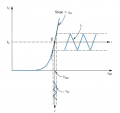# a question about the transconductance

#### Ghina Bayyat

Joined Mar 11, 2018
135
hello
i have a question about the gm of a bjt
from its defiftion gm = Δic / Δvbe and this is so clear but i don't know how did the equation turn into gm = ic / vt ???

#### MrChips

Joined Oct 2, 2009
24,232
There are two types of resistance, depending on how the resistance is measured.

1) Ohmic, DC, or static resistance
2) AC, or dynamic resistance

DC resistance R = V / I, is a measure of the resistance at a given operating voltage or current.AC resistance R = ΔV / ΔI is a measure of the rate of change of voltage and current.Conductance is the reciprocal of resistance.

Hence, conductance G = I / V

Transconductance is gm = ΔI / ΔV

The slope of the blue line in the diagram is equal to the conductance.
The slope of the red line at the given operating point is the transconductance.

Joined Mar 10, 2018
4,057
Because the small signal model is as a voltage controlled current
device -Regards, Dana

#### Ghina Bayyat

Joined Mar 11, 2018
135
thank u and i understand all of this it's not my question
i ment how is it gm = Δ ic / Δ vbe turned into gm = ic / vt
or where did vt come from and why did vbe disapear ??

#### crutschow

Joined Mar 14, 2008
27,751
how is it gm = Δ ic / Δ vbe turned into gm = ic / vt
Where did you read that this happens for a BJT?
Vt is normally used with FETs, not BJTs.

#### OBW0549

Joined Mar 2, 2015
3,566
Where did you read that this happens for a BJT?
Vt is normally used with FETs, not BJTs.
Vt has a specific meaning when discussing BJTs. It is not the same thing as Vth, the gate threshold voltage for FETs.

#### Jony130

Joined Feb 17, 2009
5,243
For the BJT the collector curtrent is described by the Shockley equation

Ic = Is * exp(Vbe/Vt)

And if we plot this equation we will get thisAnd the transconductance gm is the slope of the function Ic = f(Vbe). And in math, the slope is a differential quantity dy/dx.
For a given DC collector curremt ( Ic ) the slope of the function Ic = Is * exp(Vbe/Vt) is:

gm = d(Ic)/d(Vbe) = 1/Vt * Ie * exp(Vbe/Vt) = Ic/Vt

Where: Vt is a thermal voltage equal around 26mV

So, the gm = Ic/26mV ≈ 38.5*Ic ≈ 40*Ic

https://en.wikipedia.org/wiki/Boltzmann_constant#Role_in_semiconductor_physics:_the_thermal_voltage

Last edited:

#### Ghina Bayyat

Joined Mar 11, 2018
135
Where did you read that this happens for a BJT?
Vt is normally used with FETs, not BJTs.
Vt the thermal voltage not the threshold voltage

#### Ghina Bayyat

Joined Mar 11, 2018
135
For the BJT the collector curtrent is described by the Shockley equation

Ic = Is * exp(Vbe/Vt)

And if we plot this equation we will get this

View attachment 152721

And the transconductance gm is the slope of the function Ic = f(Vbe). And in math, the slope is a differential quantity dy/dx.
For a given DC collector curremt ( Ic ) the slope of the function Ic = Is * exp(Vbe/Vt) is:

gm = d(Ic)/d(Vbe) = 1/Vt * Ie * exp(Vbe/Vt) = Ic/Vt

Where: Vt is a thermal voltage equal around 26mV

So, the gm = Ic/26mV ≈ 38.5*Ic ≈ 40*Ic